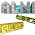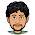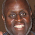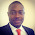## Tuesday, July 5, 2011

### Understanding Algorithm complexity, Asymptotic and Big-O notation

You'll find a lot of books and articles that cover this topic in detail for each algorithm or problem. Most of them are theoretical dealing with equations and assumptions. I am not a computational complexity theorist, so if you are one of those geeks or looking for such  material then this is not a post for you.

A programmer it absolutely required to know the cost associated with his code. So here is an attempt to simplify those complex notations and to help learn the basics.

A. Why we need it ?

Every line code or algorithm has performance or cost associated with it. We are interested in two such cost here, specifically

1. Time - also called as Time Complexity : How much time does this code take to execute ?
2. Memory - also called as Space Complexity : How much memory does this program require to execute  ?
1. Best case : What is the best case or least amount of time this code/algorithm would need to execute.
2. Worst case : What is the worst case or maximum amount of  time this code/algorithm need to execute.
3. Average case : As the name suggests it the average amount of time required to execute this code.
So we need a mechanism to compare cost of algorithms. Algorithm complexity analysis help compare cost associated with each algorithms/code.

B. What is Big-O/Asymptotic Notation ?

Simply described Big-O notation is a  function or an equation which says how much resource ( time or memory) this code needs to execute.
(it is expressed as a function of the length of its input - We'll  leave this for later * )

But why this notation ?
Its better to represent it as an equation rather than a fixed number since we have different types of computers from varying speeds, number of processor to memory etc.

Samples of Big-O notation:

Here are some sample representations of Big-O. We'll see each one of them in detail
1. O(1) - Constant time
2. O(n) - Linear time
4. O(n lg n) - Logarithmic time
How to calculate Big-O notation of a given code ?

So the fundamental question. How to find/guess the complexity of a given code ?.

Lets consider this sample code:

`int myArray[] = { 10, 6, 5, 4, 11, 7 };`

B.1. Constant time

If we want to access 3rd element in the array, we could do as

`int thirdElement = a;   // Note arrays are indexed from 0 not 1;`

Now 5th Element

`int fifthElement = a;`

So what is the cost associated with accessing an element in an array ? Its a constant time - This time remains as a constant on a given computer irrespective which element we access in the array.

Such constant time operations are represented in Big-O as

O(1) - Constant time

Where (1) is one unit of work required, The number of elements in the  array does not matter to us in this case, irrespective of length of the array the time to access any element in an array remains a constant which is represented as O(1).

B.2. Linear time

Lets consider the same above sample array:

`int myArray[] = { 10, 6, 5, 4, 11, 7 };`

Now lets say we need to print all the elements in the array, our code would look like
``` for( int i = 0; i<myArray.length; i++) {     System.out.println(myArray[i]); }```

So what is the complexity in the above case ?

Since we have 'n' elements in the array and it takes O(1) to access each element we have

O(n) - Linear time

* Now coming back to the definition where we said, Big-O is expressed as  a function of the length of its input.

In this example we can see that we have done it i.e

'n' is the number of input elements
The time complexity of this code is O(n) -

So Big-O is expressed as a function of  number of elements of the input array. This means when 'n' increases,
the time required to complete the above operation increases linearlywith respect to 'n' (input).

A single iteration (loop) over all the elements in the array gives us a complexity of O(n). If there are NO nested loops we can probably guess the complexity of the code we looking at would be in the O(n).

Lets consider the same above sample array:

`int myArray[] = { 10, 6, 5, 4, 11, 7};`

Now lets say we need to sort all the elements in the array. In this case we take each element in the array and compare it with every other element in the array

```// Sample bubble sort code. for(int i=0; i < myArray.length; i++){     for(int j=1; j < (myArray.length - i); j++){           if(myArray[j-1] > myArray[j]){            ```
```            //Swap             int temp = myArray[j-1];             myArray[j-1] = myArray[j];             myArray[j] = temp;         }     } }```

So what is the complexity in the above case ?

Since we have 'n' elements in the array and compare each element with every other element in the array, it is

O(n2)

There are nested loops in the above code/operation hence we can probably guess the complexity of the code we looking at could be in the O(n2).

A simple rule of thumb is complexity is equal to O(nx) where  x is the number of levels of loop nesting. In the above operation x = 2.

B.4. Logarithmic time

This one would be a little tricky to understand if you are new to asymptotic notations or looking at binary search for the first time.

Lets consider this sample SORTED array :

`int myArray[] = { 1, 4, 5, 6, 7, 10, 11, 25, 36 };`

Problem :
Given an element 'e' , We need to write code to see if the element is present in the array.

Eg : see if element 11 is present in the above sorted array. ( e = 11 in this case )

Binary search algorithm :

Binary search algorithm takes advantage of the fact that the array is sorted, rather than checking each element in the array from 0 to array length with the value 'e', binary search does the below
1. Take the middle element of the array
2. Compare with element 'e'
3. If middle element is equal to 'e' - We found the element
4. else if 'e' is less than middle element, The element we are looking for is in the lower half of the array - So search lower half
5. else 'e' is greater than middle element, The element we are looking for is in the upper half of the array - So search upper half
Here is the code for binary search:

```int binarySearch(int[] array, int value, int left, int right) {       if (left > right) {             return -1;       }```

```      int middle = (left + right) / 2;       if (array[middle] == value) {           return middle;       } else if (array[middle] > value) {           return binarySearch(array, value, left, middle - 1);       } else {           return binarySearch(array, value, middle + 1, right);       } }```

So what is the complexity in the above case ?

Since we have 'n' elements in the array and for each wrong guess, we discard half of the current array, so the MAXIMUM number of iterations required to find an element is

O(lg n)

Where lg =  log to the base 2.

C. What about space complexity ?

In all the above cases, to solve each of the problem we never allotted any extra space or a new array apart from the input array. Ignoring the variables we used for computation. So there were no additional space requirements for the above code.

Hence the space complexity for all the above problems is

O(1) - Constant Space

C.1. Linear Space

Here is a simple sample code that returns the copy of the input array.

```  public int[] copy(int[] array) {         int newArray[] = new int[array.length];         for (int i = 0; i < array.length; i++) {            newArray[i] = array[i];         }         return newArray;     }```

The space complexity of this code is

O(n) - Linear Space

D. Give me the list of  frequently used complexities

Here is the link : Time Complexity

E. Summary

1. Big-O notation is used to represent the cost associated with the code specifically time and memory.
2. Its an abstract mathematical representation which allows us to compare cost of the code irrespective of the type of machine/speed/memory.
3. We can compare performance of two different algorithms by just looking at the Big-O functions of these algorithms and choose which one is better for our problem in-hand.
4. Look at the levels of nesting loops in your code it helps to guess the complexity.
5. Space-time trade-off is one of the important constraints in choosing an algorithm.
F. Source code

Here is the complete sample java file used in this post.

1.nice article !

2.nice article !

3.Wow.. It's almost 6 years old but it's still useful!

1.4.5.Good one. Could have explained more why 'log n' has to be used in divide and conquer scenarios !

1.recursion doesn't always mean log (n). While doing recursion in each step, if you eliminate half of the elements, its becomes log(n) - So at every step your problem becomes - looking at only half of the elements of the original data set- So - Why log (n) ? - I'd suggest first looking at exponential, what it means - Inverse of exponential is logarthim - hope this helps

See this : https://betterexplained.com/articles/an-intuitive-guide-to-exponential-functions-e/

6.recursion doesn't always mean log (n). While doing recursion in each step, if you eliminate half of the elements, its becomes log(n) - So at every step your problem becomes - looking at only half of the elements of the original data set- So - Why log (n) ? - I'd suggest first looking at exponential, what it means - Inverse of exponential is logarthim - hope this helps

See this : https://betterexplained.com/articles/an-intuitive-guide-to-exponential-functions-e/

7.Nice and understandable

8.This comment has been removed by a blog administrator.

9.Oh! That would be better to get the explanations of the questions are discussed in here.

10.super cool answer.. very easy to understand.

11.Wonderful article, keep up the good work.

12.Bless you for this

Thanks

13.beautiful....